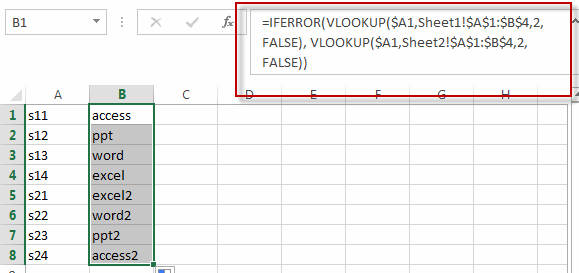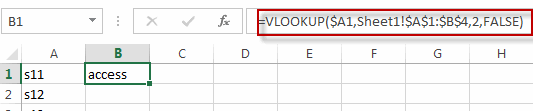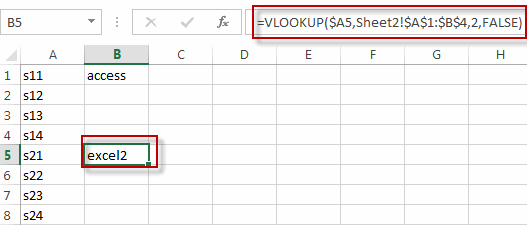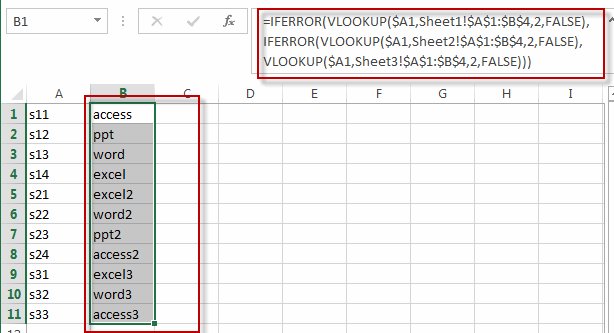# VLOOKUP Values from Multiple Worksheets

This post will guide you how to use the VLOOKUP function to find the values from multiple worksheets in Excel. For example, assuming that you have two worksheets, and you want to search the data across those two worksheet in your current worksheet (sheet3). How to do it. How to use the VLOOKUP function to search value across two or more worksheets in Excel.

You can create a new formula based on the VLOOKUP function and the IFERROR function as follows:

`=IFERROR(VLOOKUP(\$A1,Sheet1!\$A\$1:\$B\$4,2,FALSE), VLOOKUP(\$A1,Sheet2!\$A\$1:\$B\$4,2,FALSE))`Let’s see how this formula works:

=VLOOKUP(\$A1,Sheet1!\$A\$1:\$B\$4,2,FALSE)

The VLOOKUP formula will search the value of Cell A1(sheet3) in the first column in the range A1:B4 of worksheet sheet1, then return an exact match or it will return an error value #N/A.=VLOOKUP(\$A1,Sheet2!\$A\$1:\$B\$4,2,FALSE)

This formula will search the value of Cell A1(sheet3) in the first column in the range A1:B4 of another worksheet sheet2. Then it will return an exact match from column 2 that is in the same row. Or it will return the error value #N/A.=IFERROR()

This formula will check if the first VLOOKUP return an error value #N/A, if TRUE, then return the value of the second VLOOKUP function. If FALSE, then return the value of the first VLOOKUP function.

If you want to VLOOKUP values from three or more worksheet, then you can write down the following formula:

`=IFERROR(VLOOKUP(\$A1,Sheet1!\$A\$1:\$B\$4,2,FALSE), IFERROR(VLOOKUP(\$A1,Sheet2!\$A\$1:\$B\$4,2,FALSE), VLOOKUP(\$A1,Sheet3!\$A\$1:\$B\$4,2,FALSE)))`### Related Functions

• Excel VLOOKUP function
The Excel VLOOKUP function lookup a value in the first column of the table and return the value in the same row based on index_num position.The syntax of the VLOOKUP function is as below:= VLOOKUP (lookup_value, table_array, column_index_num,[range_lookup])….
• Excel IFERROR function
The Excel IFERROR function returns an alternate value you specify if a formula results in an error, or returns the result of the formula.The syntax of the IFERROR function is as below:= IFERROR (value, value_if_error)….